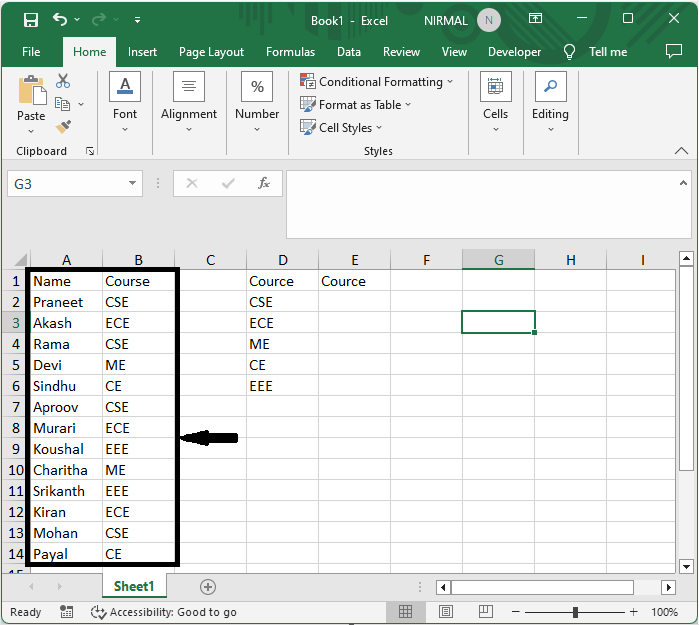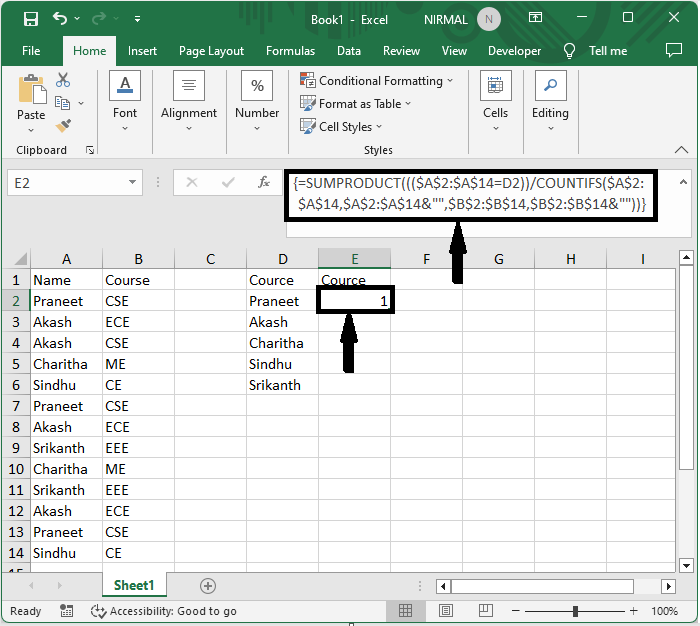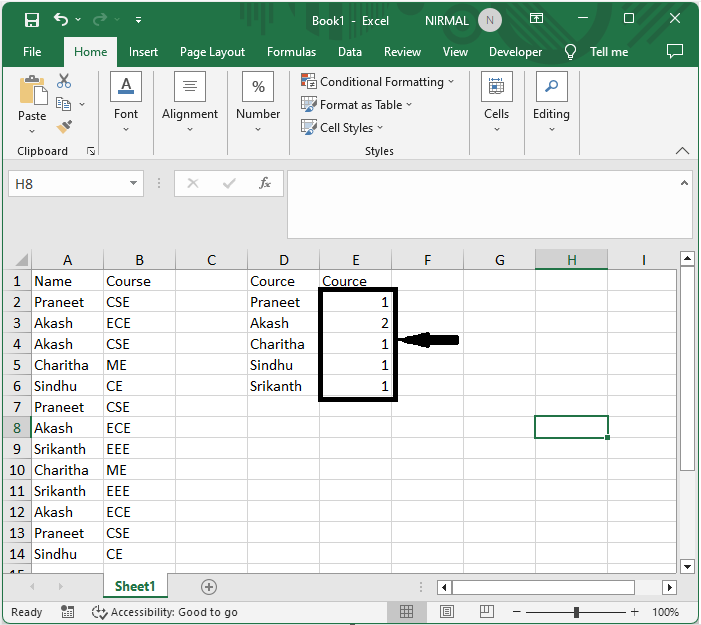# How to Count Unique Values Based on Another Column in Excel?

Excel is a powerful tool that offers various functionalities for data analysis and manipulation. One common task you might encounter is determining the number of unique values in a column, but with a condition based on another column. This tutorial will guide you through the step-by-step process of achieving this goal, enabling you to effectively analyse your data and gain valuable insights.

Throughout this tutorial, we will assume that you have a basic understanding of Excel and its functions. If you're new to Excel, don't worry! This tutorial will explain each step in detail, ensuring you can follow along and master this technique. By the end of this tutorial, you will have learned how to leverage Excel's features to count unique values based on specific conditions using a combination of functions and formulas. So, let's dive in and unlock the power of Excel for your data analysis needs!

## Count Unique Values Based on Another Column

Here we will first get the first value using the formula, then use the autofill handle to complete the task. So let us see a simple process to learn how you can count unique values based on another column in Excel.

### Step 1

Consider an Excel sheet where the data in the sheet is similar to the below image.First, click on an empty cell, in this case cell E2, and enter the formula as =SUMPRODUCT((($A$2:$A$18=D2))/COUNTIFS($A$2:$A$18,$A$2:$A$18&"",$B$2:$B$18,$B$2:$B$18&""), and click Ctrl + Shift + Enter to get the first value.

Empty Cell > Formula > Ctrl + Shift + Enter.### Step 2

Now drag down using the autofill handle to get all the values.This is how you can count unique values based on another column in Excel.

## Conclusion

In this tutorial, we have used a simple example to demonstrate how you can count unique values based on another column in Excel to highlight a particular set of data.

Updated on: 22-Aug-2023

402 Views